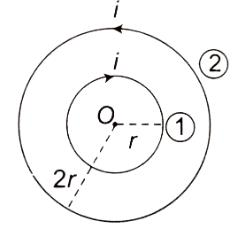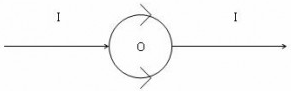Courses

# Test: Biot Savart Law

## 10 Questions MCQ Test Physics Class 12 | Test: Biot Savart Law

Description
This mock test of Test: Biot Savart Law for JEE helps you for every JEE entrance exam. This contains 10 Multiple Choice Questions for JEE Test: Biot Savart Law (mcq) to study with solutions a complete question bank. The solved questions answers in this Test: Biot Savart Law quiz give you a good mix of easy questions and tough questions. JEE students definitely take this Test: Biot Savart Law exercise for a better result in the exam. You can find other Test: Biot Savart Law extra questions, long questions & short questions for JEE on EduRev as well by searching above.
QUESTION: 1

### If we double the radius of a current carrying coil keeping the current unchanged. what happens to the magnetic field at its Centre?

Solution:

As,
B=μonI/2a
a ->radius
B ∝1/a
B1/B2=a2/a1
B1=2B2
B2=(1/2) x B1,
Magnetic field is halved.

QUESTION: 2

### A circular arc of metallic wire subtends an angle π/4 at center. If it carries current I and its radius of curvature is r, then the magnetic field at the center of the arc is

Solution:

We know,
B = μoiπ/4πR
Here,
Substituting the value of theta = π/4
So, Magnetic Field = μoIπ/π(4r x 4)r
=> B = μoI/16r

QUESTION: 3

### The magnetic field B on the axis of a circular coil at distance x far away from its centre are related as:

Solution:

dB=μoidlsin90/4πr2oidl/4πr2
Bnet= ∫dBsinθ
= ∫μoidlR/4πr2r== (μoiR/4πr3) ∫dl
= (μoiR/4πr3)2πR= μoiR2/2(R2+x2)3/2
B= μoiR2/2(R2+x2)3/2
If x>>>R
B= μoiR2/2(x2)3/2

[=B ∝ x-3]

QUESTION: 4

Two concentric coils carry the same current in opposite directions. The diameter of the inner coil is half that of the outer coil. If the magnetic field produced by the outer coil at the common centre are 1 T, the net field at the centre is

Solution:B10i/2r,
B2=μ0i/2.2r=μ0i/4r,
μ0i/4r=1T
B0=B1−B20i/4r=1T

QUESTION: 5

The magnetic field due to current element depends upon which of the following factors:

Solution:

From Biot-Savart law, magnetic field at a point p, B= (μ0​/4π)∫ [(Idl×r​)/ r3]
where r is the distance of point p from conductor and I is the current in the conductor.
Thus magnetic field due to current carrying conductor depends on the current flowing through conductor and distance from the conductor and length of the conductor.

QUESTION: 6

A circular coil of radius r carries current I. The magnetic field at its center is B. at what distance from the center on the axis of the coil magnetic field will be B/8

Solution:

B= 2μ0​i⋅πr2/4π(r2+x2)3/2
Magnetic field at centre= μ0​i/2r
B= μ0​i/2r
Put r= √3
B1=2μ0​i⋅πr2/4π(4r2)3/2
=B/8
Hence, √3R distance from the centre magnetic field is equal to magnetic field at centre

QUESTION: 7

A circular loop of radius 0.0157 m carries a current of 2 A. The magnetic field at the centre of the loop is​

Solution:

The magnetic field due to a circular loop is given by:
B= μ0​​2πi​/4πr
=10−7×2π×2/0.0157 ​
=8×10−5 Wb/m2

QUESTION: 8

A straight conductor carrying current I is split into circular loop as shown in figure a , the magnetic induction at the center of the circular loop isSolution:

The magnetic field at the center O due to the upper side of the semicircular current loop is equal and opposite to that due to the lower side of the loop.

QUESTION: 9

The magnetic field due to circular coil of 200 turns of diameter 0.1m carrying a current of 5A at a point on the axis of the coil at a distance 0.15m from the center of the coil will be

Solution:

B=μ02πnIa2/4π (a2+x2)3/2
=10−7×2×(22/7)×200×5×(0⋅1/2)2/ [(0⋅1/2)2+(0⋅15)2]3/2
=39.74x10-5

QUESTION: 10

Wire of length l, carries a steady current I. It is bent first to form a circular coil of one turn. The same wire of same length is now bent more sharply to give two loops of smaller radius the magnetic field at the centre caused by the same current is

Solution:

Let the radii be r1​ and r2​ respectively.
Since there are two turns of radius r2​, r1​=2r2​
Magnetic field B at the centre of  the coil of radius r1​ B1​=​μo​i/2r1​=​μo​i​/4r2
Magnetic field B at the center of the coil of radius r2​ B2​=2×​μo​i​/2r2
∴ B2/B1 =(2× μo​i/2r2​)/(μo​i /4r2​)​ ​​=4
Hence the answer is option C, four times its initial value.